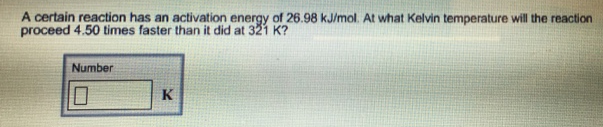Chemistry Practice Problems Arrhenius Equation Practice Problems Solution: A certain reaction has an activation energy of 26....

# Solution: A certain reaction has an activation energy of 26.98 kJ/mol. At what Kelvin temperature will the reaction proceed 4.50 times faster than it did at 321 K?

###### Problem

A certain reaction has an activation energy of 26.98 kJ/mol. At what Kelvin temperature will the reaction proceed 4.50 times faster than it did at 321 K?View Complete Written Solution

Arrhenius Equation

Arrhenius Equation

#### Q. The rate constant for the decomposition of acetaldehyde, CH3CHO, to methane, CH4, and carbon monoxide, CO, in the gas phase is 1.1 × 10−2 L/mol/s at 7...

Solved • Wed Oct 31 2018 14:37:34 GMT-0400 (EDT)

Arrhenius Equation

#### Q. The rate constant at 325 °C for the decomposition reaction C4H8 ⟶ 2C2H4 is 6.1 × 10−8 s −1, and the activation energy is 261 kJ per mole of C4H8. Dete...

Solved • Wed Oct 31 2018 14:36:07 GMT-0400 (EDT)

Arrhenius Equation

#### Q. In an experiment, a sample of NaClO3 was 90% decomposed in 48 min. Approximately how long would this decomposition have taken if the sample had been h...

Solved • Wed Oct 31 2018 14:30:52 GMT-0400 (EDT)

Arrhenius Equation

#### Q. The rate of a certain reaction doubles for every 10 °C rise in temperature.(b) How much faster does the reaction proceed at 95 °C than at 25 °C?

Solved • Wed Oct 31 2018 14:24:21 GMT-0400 (EDT)